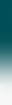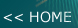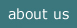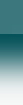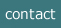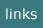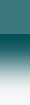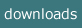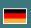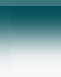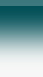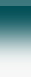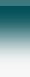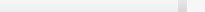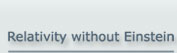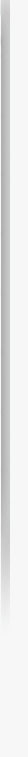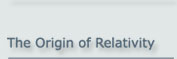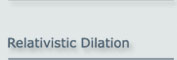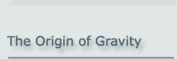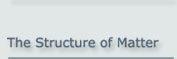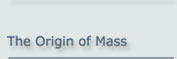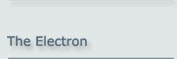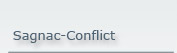Relativity without Einstein
- Relativity without Space-Time -

## It’s true! The concept of 4-dimensional space-time is by no means necessary in order to explain the physics of relativity!

Yes, relativity exists in our physical world. – But do we need Einstein?

No, we do not. And more than that: Einstein's 4-dimensional space-time is unnecessarily compli-cated and leads to logical contradictions.

The original approach to relativity as used by Hendrik A. Lorentz avoids these compli­cations and ends up with a theory which is not only much easier to understand and more closely related to physical realities, but also solves problems which are till now unresolved.

What is the difference between Einstein and Lorentz?

The fundamental difference in the starting points of Einstein and Lorentz was their assessment of the speed of light.

• To Einstein, the speed of light is truly constant in any inertial frame
• To Lorentz, the speed of light is constant with respect to only one specific frame.
• The existing measurements of the speed of light seem at the first glance compatible with Einstein's assumptions. However, the constancy of c with respect to any moving frame of reference is only apparent, because the real variations in c are obscured by changes in the moving instruments used to measure it. And Einstein's assumption can be clearly refuted by the Sagnac experiment.

Einstein based his approach to relativity on postulates, called principles. His basic postulate is the principle of relativity. It says that the physicsal laws have the same form in any intertial system. This also covers the constancy of the speed of light . This, however, is purely an assumption; the appropriate measurements do not confirm this result .

The approach of Lorentz is based on the properties of elementary particles and fields. These properties are not postulates but facts established in an independent context, namely particle physics.

The advantage which we see in the Lorentzian approach is that it is founded in physics, as well as being easier to understand and requiring a simpler mathematical treatment. This is particularly true of the processes in General Relativity, which become so simple that they can be treated at school - with similar results to the complicated reasoning of Einstein.

And it has the potential to solve problems like cosmological inflation, dark matter, and dark energy.

Now the details:

What we call RELATIVITY comprises the following measureable phenomena:

For Special Relativity:

(1)    Physical objects contract when in motion
(2)    The speed of clocks slows down in motion (and so do all temporal processes)
(3)    Mass increases - which implies mass-energy equivalence

For General Relativity:

(4)    The speed of light ‘c’ is reduced in a gravitational field
(5)    A light beam travels along a curved path in a gravitational field
(6)    The speed of clocks is slowed down in a gravitational field (and so are all temporal processes)

Einstein explained the phenomena of relativity by assuming that space and time form a close unit, which is described by the 4-dimensional geometry of “space-time”. In the general case, this 4-dimensional system is curved.

The mathematical treatment of 4-dimensional curved space is a highly challenging task. Only few physicists are able to do this.

However, the same results as those from Einstein’s space-time can be achieved using standard mathematics. And basic assumptions made by Einstein are in conflict with observations.

As a result, relativity - the special theory as well as the general theory - is not restricted to a few exceptional individuals but can be taught even at school.

>>> The goal of this website is to demonstrate this. <<<

How does this work?

Einstein’s assumptions regarding space-time can be replaced by the following facts, which exist independently of relativity:

1. The contraction is simply a consequence of the fact that the fields holding together the constituents of physical objects contract. The reason for this is the finite speed of light at which the binding fields propagate when in motion.

This consequence was noted very soon after the Michelson-Morley experiment by several physicists (e.g. Heaviside, Fitzgerald, Lorentz), who drew on Maxwell’s recently developed theory of electromagnetism. Meanwhile it has been shown that the contraction occurs for any kind of field.

2. The dilation of any periodic process (such as a clock function) in motion is a consequence of the internal oscillation of elementary particles. The constituents of elementary particles are massless and oscillate internally at the speed of light.

This oscillation inside elementary particles was basically found by Louis de Broglie in 1923 when he detected the wave properties of elementary particles. A quantitative description was given by Paul Dirac in 1928 when he developed the famous Dirac equation for the electron. Erwin Schrödinger called this motion “Zitterbewegung”.

3. The mass of elementary particles is itself also a consequence of the finite speed of light, at which the fields binding the constituents of the elementary particles propagate.

This mechanism also causes the increase in mass when an object is in motion, which is a direct consequence of the contraction. And this in turn leads directly to Einstein’s famous mass-energy equivalence equation.

For details of this process please refer to the origin of relativity and the origin of mass.

1. The reduction of the speed of light in a gravitational field is a proven fact. It can be explained by a physical model based on the assumption of exchange particles that mediate every force.

When a light-like particle interacts with the exchange particles of any force, its motion is inhibited. This causes a reduction in its effective speed which can be formally explained by this process.

2. The bending of a light-like particle in a gravitational field is a simple classical refraction process.

The stronger the gravitational field is, i.e. the closer a light-like particle is to a gravitational source, the more the speed of light is reduced. This fact causes classical refraction. The numerical result of this refraction agrees exactly with the curvature assumed by Einstein.

3. Gravitational acceleration – corresponding to gravitational mass – is the refraction of the internal motion in an elementary particle.

The oscillating constituents of an elementary particle are also refracted by the gravitational field. The elementary particle itself is thereby caused to move towards the gravitational source. This fact quantitatively explains Newton’s law of gravity in the classical case and General Relativity in the relativistic case.

For details of this process, please refer to the origin of gravity.

The conflict between Einstein's basic assumptions and actual observations

1. Einstein's assumption that the speed of light is truly constant in any frame can be disproved experimentally.

So the principle of relativity is also only an apparent fact caused by the relativistic adaption of measuring instruments. For an example, see Sagnac.

2. The (strong) equivalence principle, i.e. the equivalence of gravitation and acceleration, is falsified.

Both physical entities can occur separately in certain situations so that they can be clearly distinguished.

3. This site as pdf file.

  A. Einstein, Zur Elektrodynamik bewegter Körper, Annalen der Physik, IV. Jg. 17, S. 891–921 (1905)
  Hans Reichenbach, The Philosophy of Space & Time, Dover Publications Inc. (1958)

2022-02-17